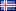ÍslenskaEnglish

27.073
4.565
1.492
2.284

# Lokaverkefni (Meistara)

Vinsamlegast notið þetta auðkenni þegar þið vitnið til verksins eða tengið í það: http://hdl.handle.net/1946/4137

Titill:
• Pluricomplex Green Functions with Logarithmic Poles at Infinity
Námsstig:
• Meistara
Höfundur:
Leiðbeinandi:
Efnisorð:
Útdráttur:
• In this thesis we study the pluricomplex Green function with logarithmic poles at
infinity, V_{X,q}, for a subset X of C^n and a weight function q on X. We study
the continuity properties of V_{X,q} with respect to X and q. If K is compact and q
continuous we show that V_{K,q} coincides with log Φ_{K,q}, where Φ_{K,q} is Siciak's extremal function.
We prove Siciak's approximation theorem for holomorphic functions, which gives
a necessary and sufficient condition for a holomorphic function to have a holomorphic
extension to a sublevel set of the Green function and the largest sublevel set where
an extension exists.
A proof of a disc formula for the Green function is presented when X is an open
domain and q upper semicontinuous. This allow us to evaluate the Green function
using analytic discs in the complex projective space P^n .

Styrktaraðili:
• Rannsóknarnámssjóður Rannís, Rannsóknasjóður Háskóla Íslands
Samþykkt:
• 18.5.2007
URI:
• http://hdl.handle.net/1946/4137

Skrár
Skráarnafn Stærð AðgangurLýsingSkráartegund
Pluricomplex_Green_Functions_fixed.pdf519.59 kBOpinnHeildartextiPDF